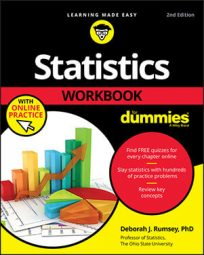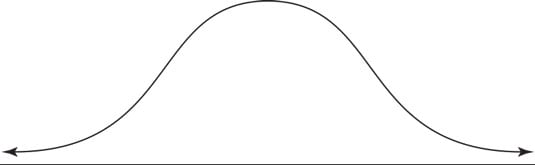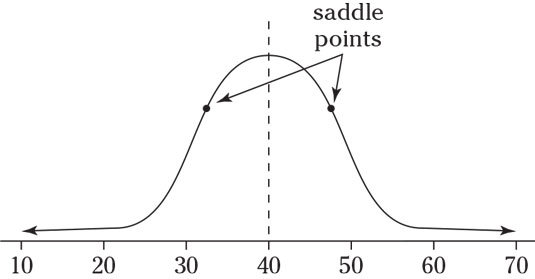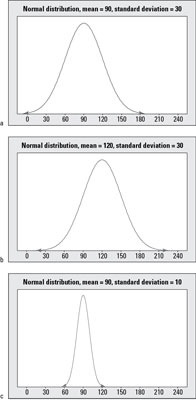##### Statistics Workbook For Dummies with Online PracticeStatisticians call a distribution with a bell-shaped curve a normal distribution. You may have heard of a bell curve. A bell curve describes data from a variable that has an infinite (or very large) number of possible values distributed among the population in a bell shape. This basically means a big group of individuals gravitate near the middle, with fewer and fewer individuals trailing off as you move away from the middle in either direction.

You can see a normal distribution’s shape in this figure.Laypeople call it a bell curve; you call it a normal distribution.

Every normal distribution has certain properties. You can use these properties to determine the relative standing of any particular result on the distribution.

When you understand the properties of the normal distribution, you'll find it easier to interpret statistical data. A continuous random variable X has a normal distribution if its values fall into a smooth (continuous) curve with a bell-shaped pattern. Each normal distribution has its own mean, denoted by the Greek letter μ and its own standard deviation, denoted by the Greek letter σ.

But no matter what their means and standard deviations are, all normal distributions have the same basic bell shape.

The properties of any normal distribution (bell curve) are as follows:

• The shape is symmetric.
• The distribution has a mound in the middle, with tails going down to the left and right.
• The mean is directly in the middle of the distribution. (The mean of the population is designated by the Greek letter μ.)
• The mean and the median are the same value because of the symmetry.
• The standard deviation is the distance from the center to the saddle point (the place where the curve changes from an “upside-down-bowl” shape to a “right-side-up-bowl” shape. (The standard deviation of the population is designated by the Greek letter σ.)• About 68 percent of the values lie within one standard deviation of the mean, about 95 percent lie within two standard deviations, and most of the values (99.7 percent or more) lie within three standard deviations by the empirical rule.
• Each normal distribution has a different mean and standard deviation that make it look a little different from the rest, yet they all have the same bell shape.

Take a look at the following figure.Three normal distributions, with means and standard deviations of a) 90 and 30; b) 120 and 30; and c) 90 and 10, respectively.
To compare and contrast the distributions shown in the figure, you first see they are all symmetric with the signature bell shape. Examples (a) and (b) have the same standard deviation, but their means are different; the mean in Example (b) is located 30 units to the right of the mean in Example (a) because its mean is 120 compared to 90. Examples (a) and (c) have the same mean (90), but Example (a) has more variability than Example (c) due to its higher standard deviation (30 compared to 10). Because of the increased variability, most of the values in Example (a) lie between 0 and 180 (approximately), while most of the values in Example (c) lie only between 60 and 120.

Finally, Examples (b) and (c) have different means and different standard deviations entirely; Example (b) has a higher mean which shifts the graph to the right, and Example (c) has a smaller standard deviation; its data values are the most concentrated around the mean.

Note that the mean and standard deviation are important in order to properly interpret numbers located on a particular normal distribution. For example, you can compare where the value 120 falls on each of the normal distributions in the above figure. In Example (a), the value 120 is one standard deviation above the mean (because the standard deviation is 30, you get 90 + 1 = 120). So on this first distribution, the value 120 is the upper value for the range where the middle 68% of the data are located, according to the Empirical Rule.

In Example (b), the value 120 lies directly on the mean, where the values are most concentrated. In Example (c), the value 120 is way out on the rightmost fringe, 3 standard deviations above the mean (because the standard deviation this time is 10, you get 90 + 3 = 120). In Example (c), values beyond 120 are very unlikely to occur because they are beyond the range where the middle 99.7% of the values should be, according to the Empirical Rule.